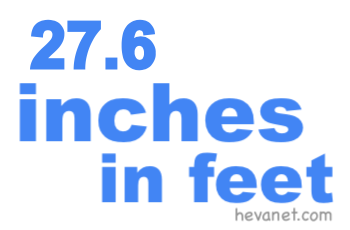27.6 inches in feetHere we will show you how to get 27.6 inches in feet (27.6 in to ft). We will show you how to convert 27.6 inches in feet as a decimal, 27.6 inches in feet and inches as a decimal, 27.6 inches in feet as a fraction, and finally, 27.6 inches in feet and inches as a fraction.

27.6 inches in feet as a decimal
We start with the easiest conversion. There are twelve inches per foot. Thus, to convert 27.6 inches to feet as a decimal, you can simply divide 27.6 by 12 like this:

27.6 ÷ 12 = 2.3
27.6 inches = 2.3 feet

27.6 inches in feet and inches as a decimal
27.6 inches makes 2 full feet per our calculation above. We subtract the full feet from 27.6 to get the remaining inches. Below is the math and the answer.

27.6 - (2 × 12) = 3.6
27.6 inches = 2 feet and 3.6 inches

27.6 inches in feet as a fraction
You already have the whole number of feet from the answer above. To get the fractional number in feet, you put the decimal inches number from the answer above over 12 and then simplify:

3.6/12 = 36/120 = 3/10
27.6 inches = 2 3/10 feet

27.6 inches in feet and inches as a fraction
Again, you already have the whole number of feet from the answer above. To get the fractional inches, you put the decimal inches number from the answer above over 1 and then simplify:

3.6/1 = 36/10 = 3 3/5
27.6 inches = 2 feet and 3 3/5 inches

Inches in Feet
Enter another length of inches below to see what it is in feet.

27.7 inches in feet
Go here for the next length in inches on our list that we have converted to feet for you.